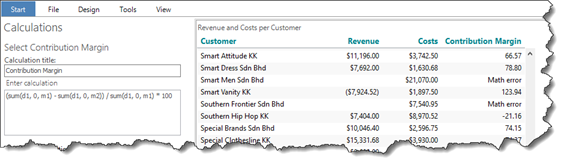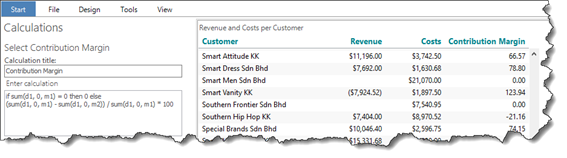# If-then-else

The demo will demonstrate a very common situation: A cross table that includes a custom calculation where a division-by-zero situation can occur.

• Create a new Analysis with a Cross table showing Revenue and Costs per Customer
• Add a new calculation Contribution Margin = (Revenue – Costs) / Revenue * 100.
• Apply the global criterion Customer Country(Territory) = Asia.Notice the “Math errors” – which are caused by division by zero.

To fix this, we will implement an “if-then-else” statement in our calculations syntax.

• In the formula, include an if-then-else statement to handle the division-by-zero issue. Insert “0” when a division by zero is attempted: